UPDATE 2020-11-10: You can find more complete detailed and optimized examples for the three following scenarios in the DAX Patterns: Currency Conversion article+video on daxpatterns.com.

Currency conversion applied to reporting can include many different scenarios.

• Data in multiple currencies, report with a single currency
• Data in multiple currencies, report with multiple currencies
• Data in a single currency, report with multiple currencies

The rule of thumb is to apply the currency exchange conversion upfront. Therefore, it is a good idea to solve all the scenarios requiring a single currency in the report by converting all amounts at the time of data import into the data model. When you need to create a report in multiple currencies, computing data in advance could be challenging and expensive. Therefore, a dynamic solution based on DAX measures and a properly designed data model makes more sense.

In this article, we only consider the third scenario, “Data in a single currency, report with multiple currencies”. The second scenario could be implemented by transforming data so that it is imported in the model in a single currency, moving the challenge over to the very scenario we describe in this article. An extended description of the three scenarios with other dynamic solutions is included in a chapter of the Analyzing Data with Microsoft Power BI and Power Pivot for Excel book.

## Introducing the data model

The data model we consider includes a Sales table with all transactions recorded in USD as a currency. There is also a hidden ExchangeRate table that contains the exchange rate for every currency in every month, assigned to the first day of each month. The AverageRate column has the value we consider the average exchange rate of the month that must be applied as a conversion rate to all the transactions included in the same month. The following diagram shows the tables that are relevant to this description.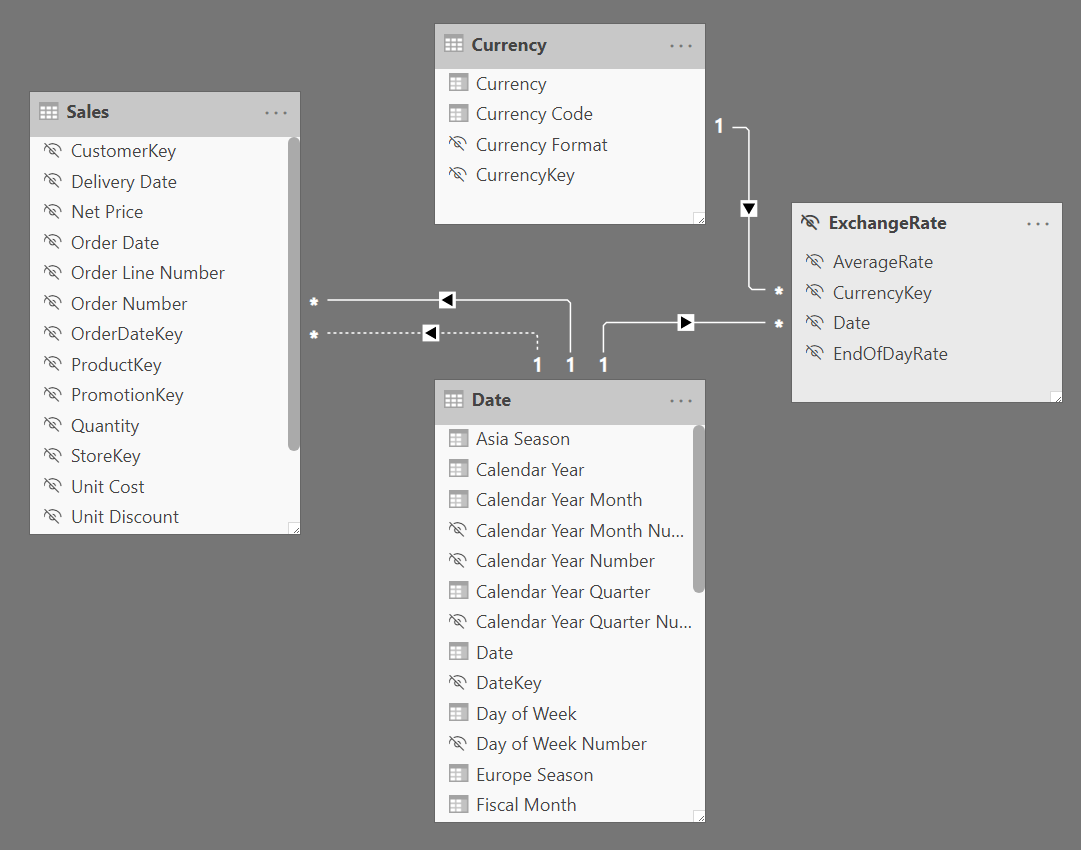The complete model also includes other tables such as Product and Customer. The following report shows the Sales Amount measure for the sales of Cell phones in 2008. All the amounts are in USD, which is the single currency used to record transactions in the Sales table.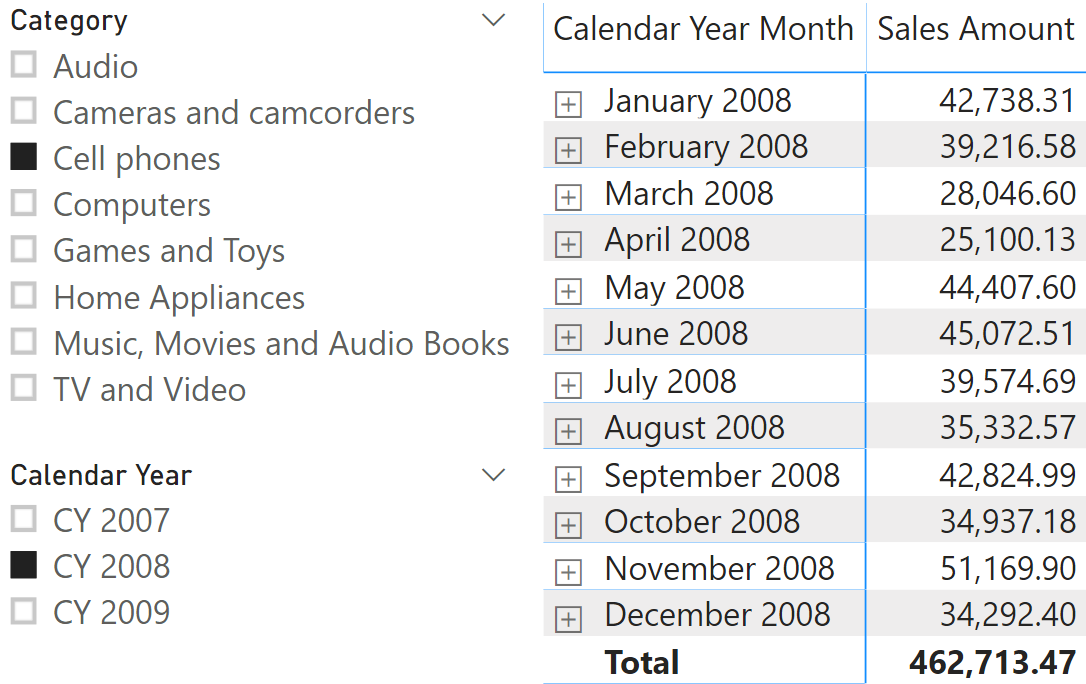The requirement is to enable the user to choose a different reporting currency by using a slicer, obtaining something like the following report where the selected reporting currency is EUR.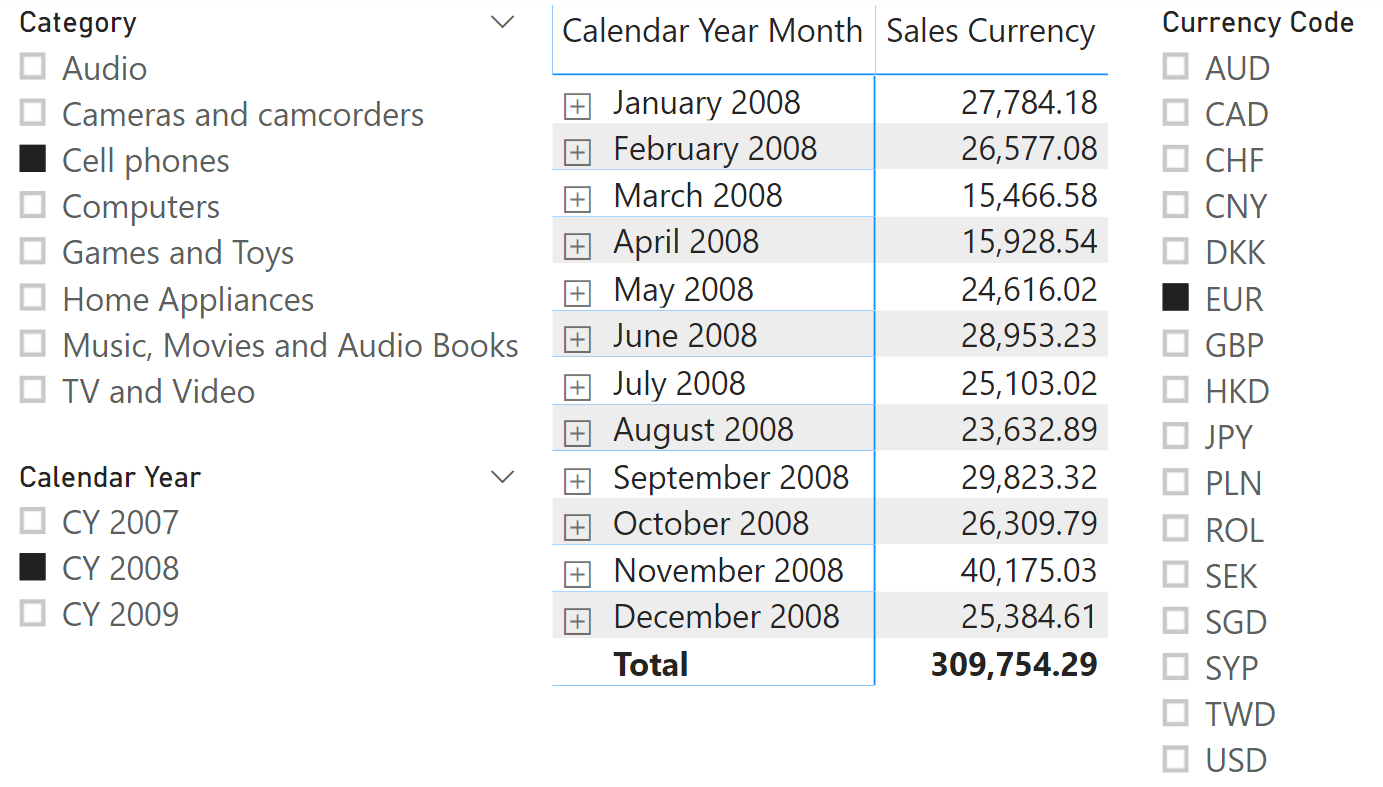## Implementing the currency conversion in DAX

The Sales Currency measure applies the required currency conversion to the result of the Sales Amount measure. In order to achieve good performance in DAX, it is better to aggregate all amounts found in the same currency and exchange rate, and then apply the conversion rate to that total, than to apply the conversion to every single transaction. Because the exchange rate is at the monthly granularity in this scenario, the Sales Currency measure is implemented by grouping Sales Amount by month, as shown in the following code:

```Sales Currency :=
IF (
ISCROSSFILTERED ( 'Currency' ),
VAR SelectedCurrency =
SELECTEDVALUE ( 'Currency'[Currency Code] )
VAR DatesExchange =
SUMMARIZE (
ExchangeRate,
'Date'[Calendar Year Month Number],
'ExchangeRate'[AverageRate]
)
VAR Result =
IF (
NOT ISBLANK ( SelectedCurrency ),
IF (
SelectedCurrency = "USD",
[Sales Amount],
SUMX (
DatesExchange,
[Sales Amount] * 'ExchangeRate'[AverageRate]
)
)
)
RETURN
Result,
[Sales Amount]
)
```

The DatesExchange variable creates a table in memory that has one row for every month. The assumption is that there is a single exchange rate for that month and a single currency selected. If this is not the case, this measure will return inaccurate numbers. It is thus important you validate the assumptions before implementing this calculation in your model.

The Result variable computes the conversion by – for each month – applying the exchange rate to the result of Sales Amount for that month. In case there is a multiple selection of currencies, the result is blank, whereas the value of Sales Amount is returned without performing any conversion in case SelectedCurrency is USD.

You might want to modify the business logic of Sales Currency to better adapt the formula to your specific requirements. For example, in case the user selects two or more currencies, the current implementation returns blank, but you might want to raise an error or display a different result in that case.

## Leveraging calculation groups

Defining the currency conversion with a specific measure like Sales Currency has two disadvantages:

1. Duplicated code and measures: The currency conversion should be applied to all the measures displaying a currency amount. If you have 3 measures (Sales Amount, Total Cost, and Margin) you have to create another three measures to display the value with the required reporting currency.
2. Format string: The format string of the result of the Sales Currency measure does not include the currency symbol. When dealing with a report with a currency selected, it could be hard to understand the currency used for the report by just looking at the numbers.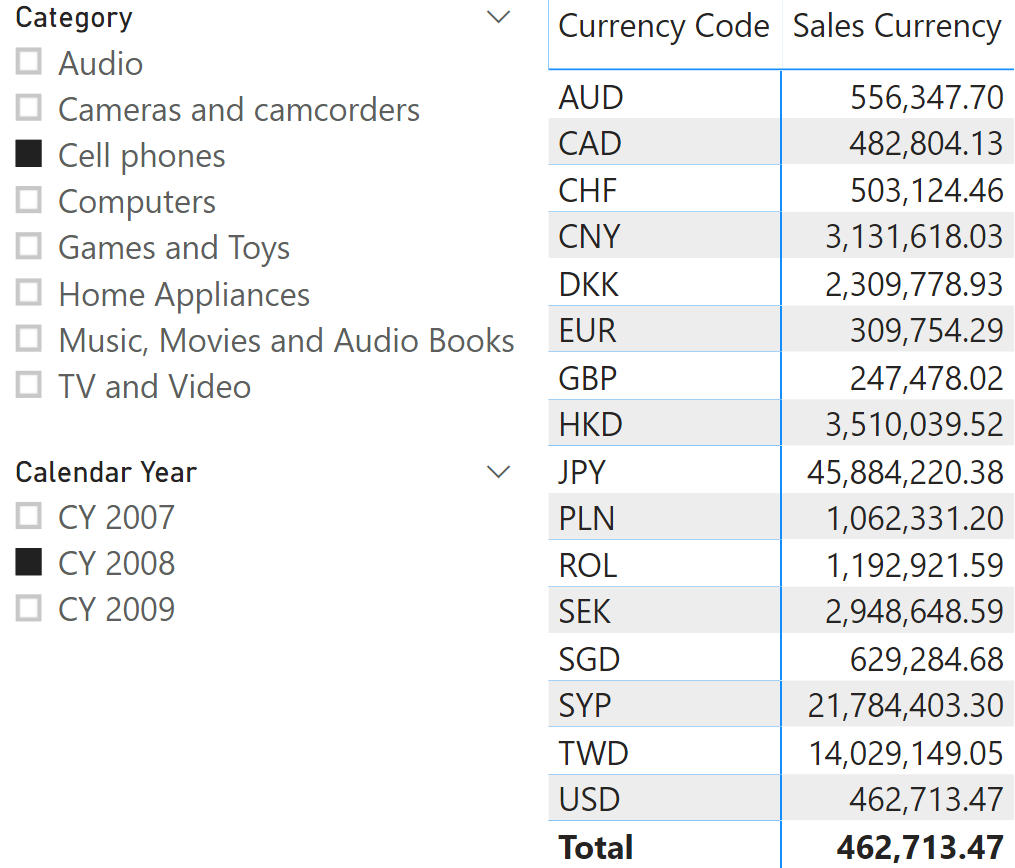By implementing the currency conversion in a calculation group, it is possible to solve both problems. At the time of writing, calculation groups are not available in Power BI. Thus, currently the remaining part of this article can only be implemented in Azure Analysis Services or Analysis Services 2019.

The expression of a calculation item that applies the currency conversion to any measure is similar to the definition of the Sales Currency measure. It just replaces the Sales Amount measure reference with a call to the SELECTEDMEASURE function:

```--
-- Calculation Group: Currency Conversion
-- Calculation Item : Report Currency
--
VAR SelectedCurrency =
SELECTEDVALUE ( 'Currency'[Currency Code], "USD" )
VAR DatesExchange =
SUMMARIZE (
ExchangeRate,
'Date'[Calendar Year Month Number]
),
"@ExchangeAverageRate", CALCULATE (
SELECTEDVALUE ( 'ExchangeRate'[AverageRate] )
)
)
VAR Result =
IF (
ISBLANK ( SelectedCurrency ) || SelectedCurrency = "USD",
SELECTEDMEASURE (),
-- Single Currency non-US selected
SUMX (
DatesExchange,
SELECTEDMEASURE () * [@ExchangeAverageRate]
)
)
RETURN
Result
```

Because this conversion could be applied to any measure, including percentages and non-currency measures such as # Quantity, it is a good practice to check whether the measure should be converted or not. In order to minimize future maintenance requirements when new measures are added to the data model, we can define a naming convention for measure names:

• # – If the measure name includes “#” then the measure represents a quantity and not a currency value. The currency conversion is not applied.
• % – If the measure name includes “%” then the measure represents a percentage and not a currency value. The currency conversion is not applied.

The calculation item definition is longer but more flexible this way:

```--
-- Calculation Group: Currency Conversion
-- Calculation Item : Report Currency
--
VAR MeasureName =
SELECTEDMEASURENAME ()
VAR SkipConversion =
NOT ISCROSSFILTERED ( 'Currency' )
|| ( SEARCH ( "#", MeasureName, 1, 0 ) > 0 )
|| ( SEARCH ( "%", MeasureName, 1, 0 ) > 0 )
RETURN
IF (
SkipConversion,
[Sales Amount],
VAR SelectedCurrency =
SELECTEDVALUE ( 'Currency'[Currency Code] )
VAR DatesExchange =
SUMMARIZE (
ExchangeRate,
'Date'[Calendar Year Month Number],
'ExchangeRate'[AverageRate]
)
VAR Result =
IF (
NOT ISBLANK ( SelectedCurrency ),
IF (
SelectedCurrency = "USD",
[Sales Amount],
SUMX (
DatesExchange,
[Sales Amount] * 'ExchangeRate'[AverageRate]
)
)
)
RETURN
Result
)
```

By using the calculation groups, it is also possible to control the format string in a dynamic way through the Format String Expression property of the calculation item. For example, the following expression uses the Currency[Currency Format] column as a format string if there is a selection on the Currency table:

```--
-- Calculation Group: Currency Conversion
-- Calculation Item : Report Currency
-- Format String Expression
--
VAR MeasureName = SELECTEDMEASURENAME()
VAR SkipConversion =
NOT ISCROSSFILTERED ( 'Currency' )
|| (SEARCH ( "#", MeasureName, 1, 0 ) > 0)
|| (SEARCH ( "%", MeasureName, 1, 0 ) > 0)
VAR CurrencyFormat =
SELECTEDVALUE ( 'Currency'[Currency Format], "#,0.00" )
VAR Result =
IF (
SkipConversion,
SELECTEDMEASUREFORMATSTRING(),
CurrencyFormat
)
RETURN
Result
```

Using the previous format string expression, we get the following result.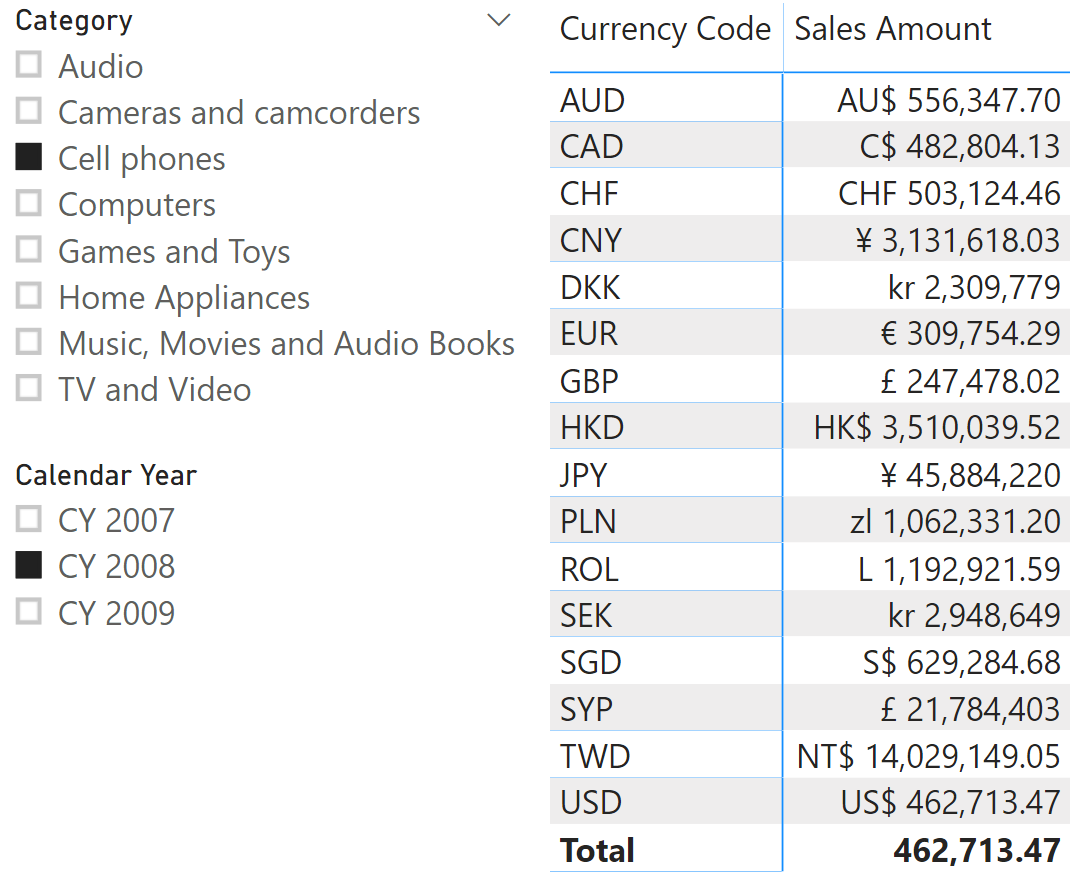## Conclusions

Currency conversion should be applied to the imported data whenever possible, thus reducing the complexity of the calculation required at query time. When it is necessary to select the reporting currency at query time, pre-calculating all the reporting currencies could be impractical, so a dynamic currency conversion can be implemented in a DAX measure. You can also implement the currency conversion in a calculation group in order to avoid the proliferation of measure (with and without currency conversion) and to dynamically modify the format string of a measure.

SELECTEDMEASUREReturns the measure that is currently being evaluated.

`SELECTEDMEASURE ( )`